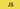# Currying in Javascript - Javascript weeklyIn this article, we will see what is currying and why we need it. Currying in Javascript - Javascript weekly

### What is Currying in Javascript?

Currying in Javascript is nothing but changing a function with multiple arguments to a function which takes only one argument.

you can ask me, how it's possible to do?. let's try to understand this concept with an example.

`1let getUser = (firstname, lastname, age) => {2  console.log(3    "Hi, My Name is " + firstname + " " + lastname + " and Age is " + age4  )5}67getUser("John", "Sonmez", 25)`

consider the above function which takes multiple arguments and print the values in the output

Instead of doing it that way, we can curry the function and takes the argument one by one.

For Example,

`1function getuserCurry(firstname) {2  return function(lastname) {3    return function(age) {4      console.log(5        "Hi, My Name is " + firstname + " " + lastname + " and Age is " + age6      )7    }8  }9}1011getuserCurry("Curry")("Function")(22)`

In the above function, we take one argument at a time and return a function which takes an another argument. it goes on until there is no argument to pass.

curry functions retains the state of the variable in it. it is called as Closure in javascript.

That's cool. But, why do we need currying in Javascript if we can do that in multiple arguments.

Let's understand this with a real time use case.

### Currying - real time use case

Let's say that you fetch a data something like this

`1let users = [2  {3    id: 1,4    name: "John",5    age: 22,6  },7  {8    id: 2,9    name: "Peters",10    age: 23,11  },12  {13    id: 3,14    name: "Smith",15    age: 24,16  },17  {18    id: 4,19    name: "Woakes",20    age: 25,21  },22]`

In the above data, you need to fetch id from the array of objects. you can simply use map function to simply interate over it and get the id's from it

`1users = users.map(item => item.id)`

it will return an array of ids. what happens if we need to do the same functionality again for another array of objects

`1let names = [2  {3    id: 5,4    name: "dfdsfds",5    age: 22,6  },7  {8    id: 6,9    name: "ewrwer",10    age: 23,11  },12  {13    id: 7,14    name: "retretret",15    age: 24,16  },17  {18    id: 8,19    name: "tyryt",20    age: 25,21  },22]`

you need to do the same loop and get only the ids from it. you can simplify this way by using curry

`1const get = function(property) {2  return function(object) {3    return object[property]4  }5}`

Define a function which takes one argument at time and return a function inside of it which takes an another argument.(which is basically a closure).

Now, you need to call the function get() which will return a function.

`1const getId = get("id")`

After that, you need to pass the function inside the map loop which will get you all the id's

`1let userids = users.map(getId)23console.log("userids", userids)`## Cloud Native Web Development

A Hands-On Guidebook to Learn Cloud Native Web Development - From Zero To Production

it will print output something like this

Now, you can run the following function to the second array of object. no need to rewrite again.

`1const namelist = names.map(getId)23console.log("namelist", namelist)`

output will be

That's one the real time use cases where you can apply the curry in javascript.

### Currying Use Cases

• Function Composition - function composition is an important concept in javascript which needs a complete post. In simple terms, it is a way to combine two or more functions to return the result. For Ex, result = a( b(x) ) where b(x) is a function which returns a result.Further, the result will be passed as a argument to a function.
• First-class function - you can be able to create a first class function. first class function is nothing but a function can be passed as an argument.

currying is an important concept in Functional programming paradigms. it is used along with Partial application and Function Composition.

we will see what are Partial Application and Function Composition in upcoming articles.

Until then, Happy Coding :-)

If you want to learn Functional Programming for JavaScript.

you can read the Kyle Simpson Functional Programming book which is a great book . Also,

You Don′t Know JS – Scope and Closures